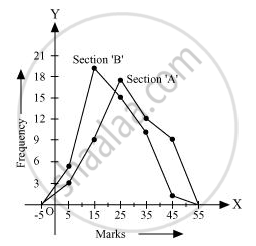Share

# The Following Table Gives the Distribution of Students of Two Sections According to the Mark Obtained by Them - CBSE Class 9 - Mathematics

ConceptGraphical Representation of Data

#### Question

The following table gives the distribution of students of two sections according to the mark obtained by them:-

 Section A Section B Marks Frequency Marks Frequency 0 - 10 3 0 - 10 5 10 - 20 9 10 - 20 19 20 - 30 17 20 - 30 15 30 - 40 12 30 - 40 10 40 - 50 9 40 - 50 1

Represent the marks of the students of both the sections on the same graph by two frequency polygons. From the two polygons compare the performance of the two sections.

#### Solution

We can find the class marks of the given class intervals by using the following formula.

"Class mark"="Upper class limit + Lower class limit"/2

 Section A Section B Marks Class marks Frequency Marks Class marks Frequency 0 − 10 5 3 0 − 10 5 5 10 − 20 15 9 10 − 20 15 19 20 − 30 25 17 20 − 30 25 15 30 − 40 35 12 30 − 40 35 10 40 − 50 45 9 40 − 50 45 1

Taking class marks on x-axis and frequency on y-axis and choosing an appropriate scale (1 unit = 3 for y-axis), the frequency polygon can be drawn as follows.It can be observed that the performance of students of section ‘A’ is better than the students of section ‘B’ in terms of good marks.

Is there an error in this question or solution?

#### APPEARS IN

NCERT Solution for Mathematics Class 9 (2018 to Current)
Chapter 14: Statistics
Ex. 14.30 | Q: 6 | Page no. 260

#### Video TutorialsVIEW ALL 

Solution The Following Table Gives the Distribution of Students of Two Sections According to the Mark Obtained by Them Concept: Graphical Representation of Data.
S